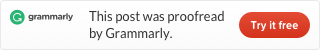# LeetCode problem 567 Permutation in String (Java)

Given two strings s1 and s2, write a function to return true if s2 contains the permutation of s1. In other words, one of the first string’s permutations is the substring of the second string.

Example 1:

Input:
s1 = “ab”
s2 = “eidbaooo”
Output: True
Explanation: s2 contains one permutation of s1 (“ba”).

Example 2:

Input:
s1= “ab”
s2 = “eidboaoo”
Output: False

Note:

1. The input strings only contain lower case letters.
2. The length of both given strings is in the range [1, 10,000].

After reading the request, you will find out this problem is almost the same as problem 438. The word permutation is the same as the word anagrams. So it is easy, we can just use function findAnagrams from problem 438 to solve this problem.